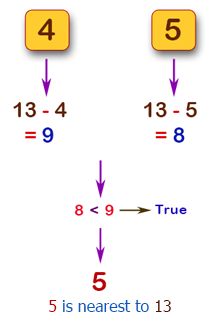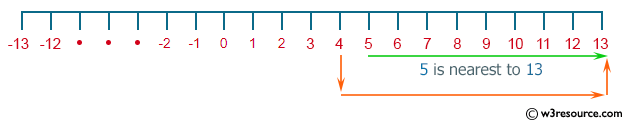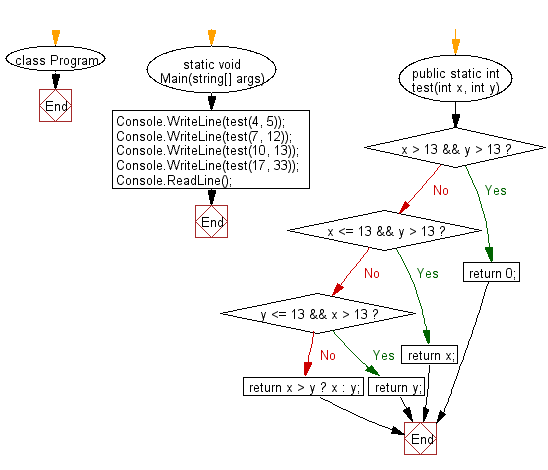﻿ C# - Find the value nearest to 13 from two given integers# C# Sharp Basic Algorithm Exercises: Check two given integers and return the value whichever value is nearest to 13 without going over

## C# Sharp Basic Algorithm: Exercise-58 with Solution

Write a C# Sharp program to check two given integers and return the value whichever value is nearest to 13 without going over. Return 0 if both numbers go over.

Pictorial Presentation:Sample Solution:-

C# Sharp Code:

``````using System;
using System.Collections.Generic;
using System.Linq;
using System.Text;
namespace exercises
{
class Program
{
static void Main(string[] args)
{
Console.WriteLine(test(4, 5));
Console.WriteLine(test(7, 12));
Console.WriteLine(test(10, 13));
Console.WriteLine(test(17, 33));
}
public static int test(int x, int y)
{
if (x > 13 && y > 13) return 0;
if (x <= 13 && y > 13) return x;
if (y <= 13 && x > 13) return y;
return x > y ? x : y;
}
}
}
```
```

Sample Output:

```5
12
13
0
```

Flowchart:C# Sharp Code Editor:

Improve this sample solution and post your code through Disqus

What is the difficulty level of this exercise?

Test your Programming skills with w3resource's quiz.

﻿